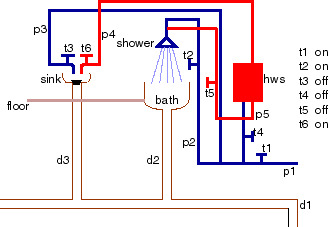CS502 Fall 2006
Assignment 3

Due: 12:30pm, Monday 16 October 2006.

# Question 1

Consider the language with constant symbols a, b and c, predicate symbols p, q, and r, and the knowledge base KB that consists of the clauses:
```p(X) <- q(X).
p(Y) <- r(Y).
q(a).
r(b).
```
Suppose there are two individuals fred and mary.
1. Give a model of KB where D={fred,mary}. You must specify phi and pi.
2. Give an interpretation with the same domain that isn't a model of KB. You must specify phi and pi.
3. Give three atoms that are logical consequences of the knowledge base.
4. Give three atoms that are not logical consequences of the knowledge base.

# Question 2

Consider the domain of house plumbing represented below.

In this example, constants p1, p2 and p3 denote cold water pipes. p1 is the pipe coming in from the main water supply. Constants p4 and p5 represent hot water pipes (coming out from the hot water system). Constants t1, t2 t3, t4 and t5 denote taps and d1, d2 and d3 denote drainage pipes. The constants shower denotes a shower, bath denotes a bath, sink denotes a sink, hws denotes a hot water system, and floor denotes the floor. The diagram below is intended to give the denotation for the constant symbols.plumbing2.gif

The Plumbing Domain

Suppose we have as predicate symbols:

• pressurised, where pressurised(P) is true if pipe P has mains pressure in it. p1 is always pressurized. Other pipes are pressurized if they are connected to a pressurized pipe through an open tap.
• on, where on(T) is true if tap T is on.
• off, where off(T) is true if tap T is off.
• wet, where wet(B) is true if B is wet.
• flow, where flow(P) is true if water is flowing through P.
• plugged, where plugged(S) is true if S is either a sink or a bath and has the plug in.
• unplugged, where unplugged(S) is true if S is either a sink or a bath and doesn't have the plug in.
Assume that the taps and plugs have been in the same positions for one hour; you don't need to consider the dynamics of turning on taps and inserting and removing plugs.

The file plumbingbuggy.pl contains a CILog axiomatization for the case where tap t1 is on, t2 is on, t3 is off, t4 is off, t5 is off, and t6 is on. There is a plug in the sink, but not in the bath. (This has been formatted to be unreadable, but you don't need to be able to read the program, or know how it is implemented, to be able to debug it.)

Unfortunately there is a bug in the program. We know this because we can prove that the floor is wet, even though it isn't wet in the intended interpretation. Using CILog, your goal is to find a clause that is false in the intended interpretation, using just the intended interpretation of the symbols.

You need to hand in a trace of the CILog session, and show clearly which is the buggy rule.

# Question 3

It is often a problem to determine where a document you printed actually got printed. It can depend on which room you selected the output to go to and which printer is plugged into the printer port in that room.

Suppose there are three computers, named c1, c2 and c3, two rooms, r101 and r202, and three printers pr1, pr2 and pr3. Each computer has a room selected. Each room can have a printer plugged into its port.

Using CILog, we want to axiomatize a domain so that you can answer questions about which room a document was printed and which printer it was printed on.

1. Give the intended interpretation of each symbol used.
2. Give the general axioms that are true for all configurations of printers.
3. Show that your axiomatization works for more than one configuration.

# Question 4

Using one of the OWL editors (e.g., Protégé or SWOOP), write an ontology in OWL about buildings, that includes at least the following concepts:

Building, residential building, house, apartment building, condominium building, duplex, townhouse, owner-occupied, rental, multi-family dwelling, commercial building, mixed commercial-residential building.

# Question 5

For each question in this assignment, say how long you spent on it. Was this reasonable? What did you learn?
David Poole